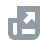Pay with
Markets
NFT
New
English
USDAccount FunctionsTutorialBinance Fan TokenBinance EarnCrypto Deposit/WithdrawalCrypto Derivatives
Futures Contracts
Introduction to Binance Futures
USDⓈ-M Futures Contracts
COIN-M Futures Contracts
Futures Guide
Cross Collateral
Hedge Mode
Types of Orders
Binance Futures Welcome Bonus Coupon
Futures Service Agreement
Binance Futures Related Programs
Index
Options
Leveraged TokensFinanceAPISecurityNFTVIP

# How to Calculate Profit and Loss for Futures Contracts

2021-07-28 07:06
Profit and loss calculations are based on the contract’s collateral. For instance, a USDⓈ-margined contract will be denominated in USDT. Whereas, BTC Coin-margined contracts will be denominated in BTC.
Do note that unrealized Profit and Loss are calculated based on the Mark Price, while realized Profit and Loss are calculated based on the last price.

## Profit and Loss calculations for Coin-margined contracts (BTCUSD)

A BTC coin-margined contract is denominated, collateralized and settled in Bitcoin, which means Bitcoin is used as the base currency. Each BTC coin-margined contract represents 100 USD and as such, USD is the counter currency. Since each contract represents a fixed quantity of USD, this means Bitcoin is used to fund the initial margin or calculate profit and loss.
Assume you purchased 100 Bitcoin-margined perpetual contracts (100 x 100 USD = \$10,000) at \$50,000 each. By doing this, you are essentially selling USD 10,000 and buying an equivalent value of Bitcoin (10,000/50,000 = 0.2 BTC).
Suppose Bitcoin’s price rose to \$55,000, and you want to secure profits from the trade. To close the position, you buy back USD 10,000 worth of contracts and simultaneously sell the equivalent of Bitcoin (10,000/55,000 = 0.1818 BTC).
In this trade, your profit will be calculated as such: Quantity of Bitcoins at Entry - Quantity of Bitcoins at Exit = 0.2 - 0.1818 = 0.0182 BTC.
In short, the profit and loss formula will be:
((1 / Futures Entry Price) - (1 / Futures Exit Price)) * Position Size
((1/50,000) - (1/55,000)) * (100 contracts x 100USD) = 0.0182 BTC
Example for short position:
Short BTCUSD 0925 Quarterly (Buy USD, Sell BTC):
((1 / Futures Entry Price) - (1 / Futures Exit Price)) * (Position Size * -1)
((1/50,000) - (1/45,500)) * (100 contracts x 100USD) = 0.0198 BTC

## Profit and Loss calculations for USDⓈ-margined contracts (BTCUSDT)

Assume you purchase (long) 10,000 USDT worth of BTCUSDT perpetual contracts at 50,000 USDT. The price increases and you exit at 55,000 USDT. Your profit will be:
((1 / Futures Entry Price) - (1 / Futures Exit Price)) * Position Size
( 1 / 50,000 - 1 / 55,000 ) * 10,000 = 0.018182 Bitcoin
Conversion to USDT = 0.018182 * 55,000 USDT = 1,000 USDT
Assume you sold (short) 10,000 USDT worth of BTCUSDT perpetual contracts at 50,000 USDT. The price decreases and you exit at 45,000 USDT. Your profit will be:
((1 / Futures Entry Price) - (1 / Futures Exit Price)) * (Position Size * -1)
( 1 / 50,000 - 1 / 45,000 ) * -10,000 = 0.022 Bitcoin
Conversion to USDT = 0.022 * 45,000 USDT = 1,000 USDT

## How to calculate Unrealized PNL and ROE%

### USDⓈ-M Futures Contracts

• Users choose Mark price as price basis:
Unrealized PNL = position size * direction of order * (mark price - entry price)
ROE% =Unrealized PNL in USDT / entry margin = ( ( mark Price - entry Price ) * direction of order * size ) / （position_amount * contract_multiplier * mark_price* IMR）
*IMR = 1/Leverage
• Users choose Latest price as price basis:
Unrealized PNL = position size * direction of order * (latest price - entry price)
ROE% = Unrealized PNL in USDT / entry margin = ( ( latest price - entry Price ) * direction of order * size ) / （position_amount * contract_multiplier * mark_price* IMR）
direction of order: 1 for long order；-1 for short order

### COIN-M Futures Contracts

• Users choose Mark price as price basis:
Unrealized PNL = position_size * contract_multiplier * direction of order * (1 / entry price - 1 / mark price)
ROE% = Unrealized PNL * mark price / abs(size) * contract_multiplier * IMR
• Users choose Latest price as price basis:
Unrealized PNL = position_size * contract_multiplier * direction of order * (1 / entry price - 1 / latest price)
ROE% = Unrealized PNL * mark_price / abs(size) * contract_multiplier * IMR